# Time Series Anomaly Detection Using Deep Learning

This example shows how to detect anomalies in sequence or time series data.

To detect anomalies or anomalous regions in a collection of sequences or time series data, you can use an autoencoder. An autoencoder is a type of model that is trained to replicate its input by transforming the input to a lower dimensional space (the encoding step) and reconstructing the input from the lower dimensional representation (the decoding step). Training an autoencoder does not require labeled data.

An autoencoder itself does not detect anomalies. Training an autoencoder using only representative data yields a model that can reconstruct its input data by using features learned from the representative data only. To check if an observation is anomalous using an autoencoder, input the observation into the network and measure the error between the original observation and the reconstructed observation. A large error between the original and reconstructed observations indicates that the original observation contains features unrepresentative of the data used to train the autoencoder and is anomalous. By observing the element-wise error between the original and reconstructed sequences, you can identify localized regions of anomalies.

This image shows an example sequence with anomalous regions highlighted.This example uses the Waveform data set which contains 2000 synthetically generated waveforms of varying length with three channels.

Load the Waveform data set from `WaveformData.mat`. The observations are `numChannels`-by-`numTimeSteps` arrays, where `numChannels` and `numTimeSteps` are the number of channels and time steps of the sequence, respectively.

`load WaveformData`

View the sizes of the first few sequences.

`data(1:5)`
```ans=5×1 cell array {3×103 double} {3×136 double} {3×140 double} {3×124 double} {3×127 double} ```

View the number of channels. To train the network, each sequence must have the same number of channels.

`numChannels = size(data{1},1)`
```numChannels = 3 ```

Visualize the first few sequences in a plot.

```figure tiledlayout(2,2) for i = 1:4 nexttile stackedplot(data{i}',DisplayLabels="Channel " + (1:numChannels)); title("Observation " + i) xlabel("Time Step") end```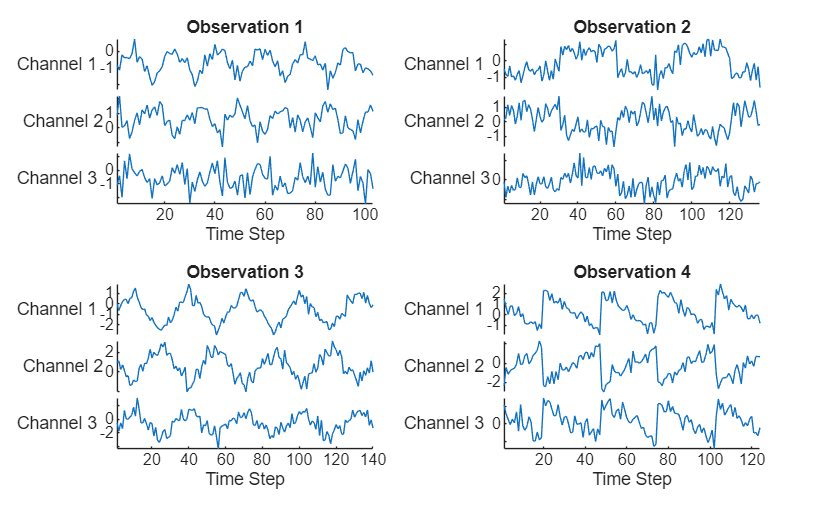Partition the data into training and validation partitions. Train the network using the 90% of the data and set aside 10% for validation.

```numObservations = numel(data); XTrain = data(1:floor(0.9*numObservations)); XValidation = data(floor(0.9*numObservations)+1:end);```

### Prepare Data for Training

The network created in this example repeatedly downsamples the time dimension of the data by a factor of two, then upsamples the output by a factor of two the same number of times. To ensure that the network can unambiguously reconstruct the sequences to have the same length as the input, truncate the sequences to have a length of the nearest multiple of ${2}^{K}$, where $K$ is the number of downsampling operations.

Downsample the input data twice.

`numDownsamples = 2;`

Truncate the sequences to the nearest multiple of `2^numDownsamples`. So that you can calculate the minimum sequence length for the network input layer, also create a vector containing the sequence lengths.

```sequenceLengths = zeros(1,numel(XTrain)); for n = 1:numel(XTrain) X = XTrain{n}; cropping = mod(size(X,2), 2^numDownsamples); X(:,end-cropping+1:end) = []; XTrain{n} = X; sequenceLengths(n) = size(X,2); end```

Truncate the validation data using the same steps.

```for n = 1:numel(XValidation) X = XValidation{n}; cropping = mod(size(X,2),2^numDownsamples); X(:,end-cropping+1:end) = []; XValidation{n} = X; end```

### Define Network Architecture

Define the following network architecture, which reconstructs the input by downsampling and upsampling the data.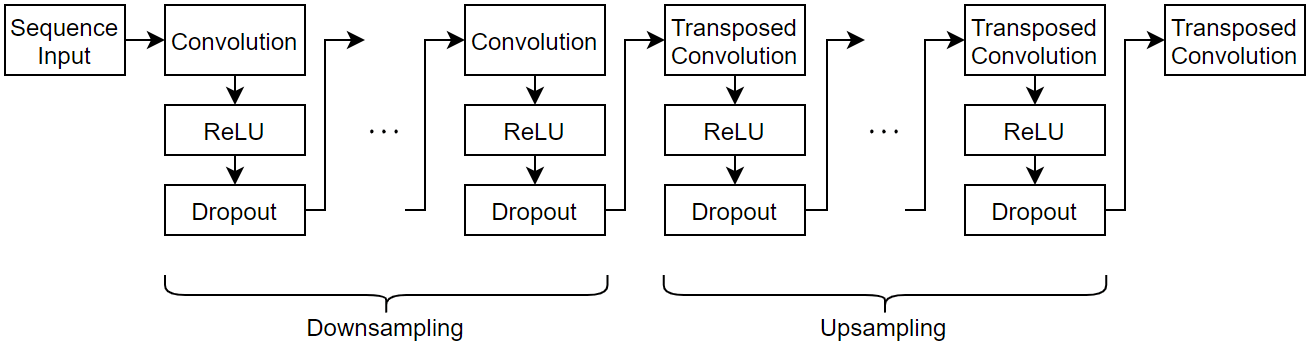• For sequence input, specify a sequence input layer with an input size matching the number of input channels. Normalize the data using Z-score normalization. To ensure that the network supports the training data, set the `MinLength` option to the length of the shortest sequence in the training data.

• To downsample the input, specify repeating blocks of 1-D convolution, ReLU, and dropout layers. To upsample the encoded input, include the same number of blocks of 1-D transposed convolution, ReLU, and dropout layers.

• For the convolution layers, specify decreasing numbers of filters with size 7. To ensure that the outputs are downsampled evenly by a factor of 2, specify a stride of `2`, and set the `Padding` option to `"same"`.

• For the transposed convolution layers, specify increasing numbers of filters with size 7. To ensure that the outputs are upsampled evenly be a factor of 2, specify a stride of `2`, and set the `Cropping` option to `"same"`.

• For the dropout layers, specify a dropout probability of 0.2.

• To output sequences with the same number of channels as the input, specify a 1-D transposed convolution layer with a number of filters matching the number of channels of the input. To ensure output sequences are the same length as the layer input, set the `Cropping` option to `"same"`.

• Finally, include a regression layer.

To increase or decrease the number of downsampling and upsampling layers, adjust the value of the `numDownsamples` variable defined in the Prepare Data for Training section.

```minLength = min(sequenceLengths); filterSize = 7; numFilters = 16; dropoutProb = 0.2; layers = sequenceInputLayer(numChannels,Normalization="zscore",MinLength=minLength); for i = 1:numDownsamples layers = [ layers convolution1dLayer(filterSize,(numDownsamples+1-i)*numFilters,Padding="same",Stride=2) reluLayer dropoutLayer(dropoutProb)]; end for i = 1:numDownsamples layers = [ layers transposedConv1dLayer(filterSize,i*numFilters,Cropping="same",Stride=2) reluLayer dropoutLayer(dropoutProb)]; end layers = [ layers transposedConv1dLayer(filterSize,numChannels,Cropping="same") regressionLayer];```

To interactively view or edit the network, you can use Deep Network Designer.

```deepNetworkDesigner(layers) ```

### Specify Training Options

Specify the training options:

• Train using the Adam solver.

• Train for 120 epochs.

• Shuffle the data every epoch.

• Validate the network using the validation data. Specify the sequences as both the inputs and the targets.

• Display the training progress in a plot.

• Suppress the verbose output.

```options = trainingOptions("adam", ... MaxEpochs=120, ... Shuffle="every-epoch", ... ValidationData={XValidation,XValidation}, ... Verbose=0, ... Plots="training-progress");```

### Train Network

Train the network using the `trainNetwork` function. When you train an autoencoder, the inputs and targets are the same. Specify the training data as both the inputs and the targets.

`net = trainNetwork(XTrain,XTrain,layers,options);`

### Test Network

Test the network using the validation data. For each validation sequence, calculate the mean absolute error (MAE) between the sequence and the reconstructed sequence.

```YValidation = predict(net,XValidation); MAEValidation = zeros(numel(XValidation),1); for n = 1:numel(XValidation) X = XValidation{n}; Y = YValidation{n}; MAEValidation(n) = mean(abs(Y - X),'all'); end```

Visualize the MAE values in a histogram.

```figure histogram(MAEValidation) xlabel("Mean Absolute Error (MAE)") ylabel("Frequency") title("Representative Samples")```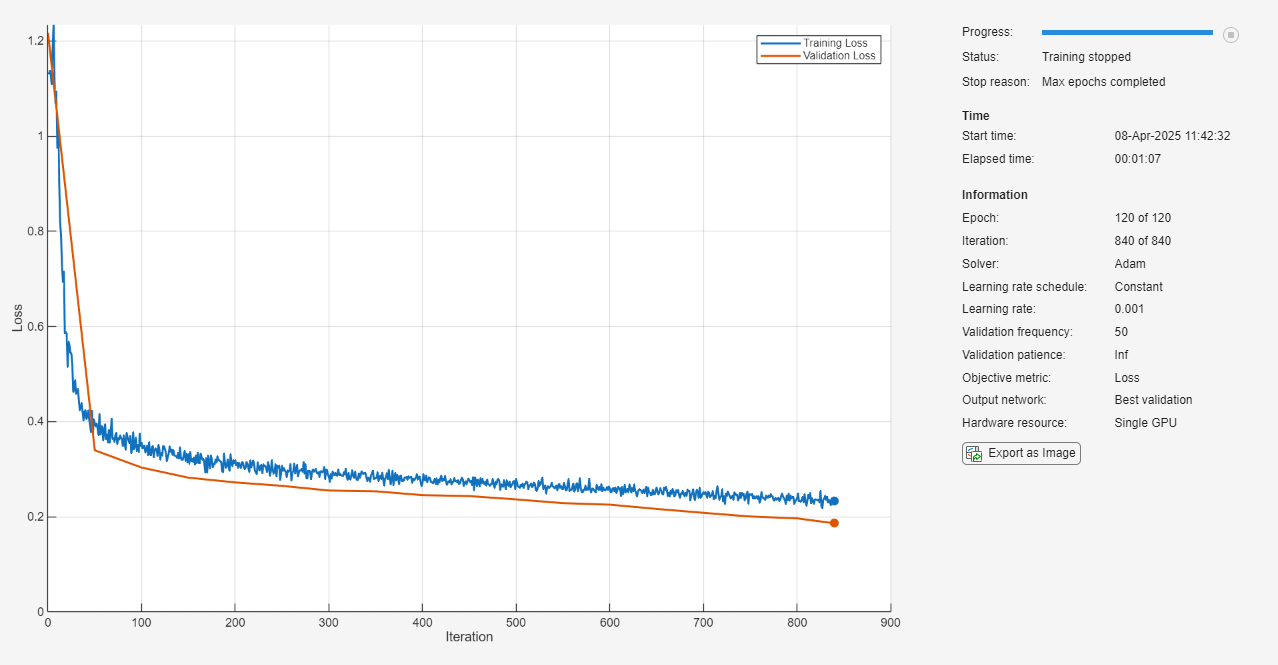You can use the maximum MAE as a baseline for anomaly detection. Determine the maximum MAE from the validation data.

`MAEbaseline = max(MAEValidation)`
```MAEbaseline = 0.4895 ```

### Identify Anomalous Sequences

Create a new set of data by manually editing some of the validation sequences to have anomalous regions.

Create a copy of the validation data.

`XNew = XValidation;`

Randomly select 20 of the sequences to modify.

```numAnomalousSequences = 20; idx = randperm(numel(XValidation),numAnomalousSequences);```

For each of the selected sequences, set a patch of the data `XPatch` to `4*abs(Xpatch)`.

```for i = 1:numAnomalousSequences X = XNew{idx(i)}; idxPatch = 50:60; XPatch = X(:,idxPatch); X(:,idxPatch) = 4*abs(XPatch); XNew{idx(i)} = X; end```

Make predictions on the new data.

`YNew = predict(net,XNew);`

For each prediction, calculate the MAE between the input sequence and the reconstructed sequence.

```MAENew = zeros(numel(XNew),1); for n = 1:numel(XNew) X = XNew{n}; Y = YNew{n}; MAENew(n) = mean(abs(Y - X),'all'); end```

Visualize the MAE values in a plot.

```figure histogram(MAENew) xlabel("Mean Absolute Error (MAE)") ylabel("Frequency") title("New Samples") hold on xline(MAEbaseline,"r--") legend(["Data" "Baseline MAE"])```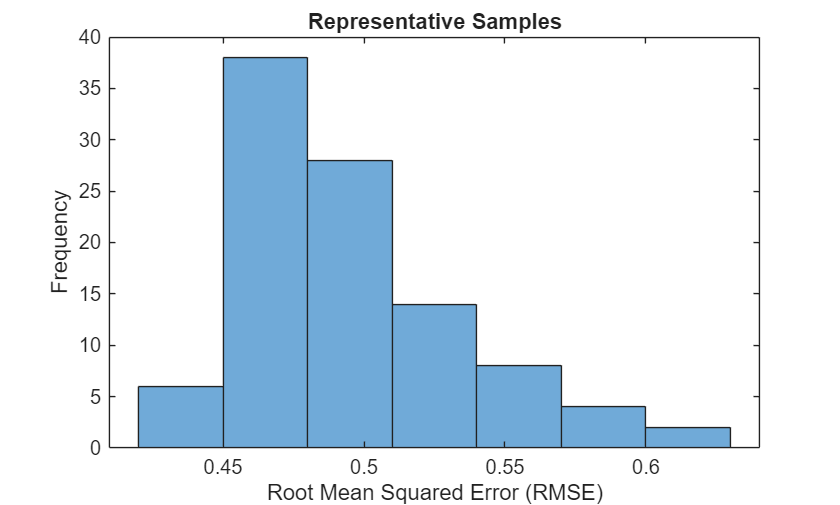Identify the top 10 sequences with the largest MAE values.

```[~,idxTop] = sort(MAENew,"descend"); idxTop(1:10)```
```ans = 10×1 99 31 39 56 23 95 35 36 3 54 ```

Visualize the sequence with the largest MAE value and its reconstruction in a plot.

```X = XNew{idxTop(1)}; Y = YNew{idxTop(1)}; figure t = tiledlayout(numChannels,1); title(t,"Sequence " + idxTop(1)) for i = 1:numChannels nexttile plot(X(i,:)) box off ylabel("Channel " + i) hold on plot(Y(i,:),"--") end nexttile(1) legend(["Original" "Reconstructed"])```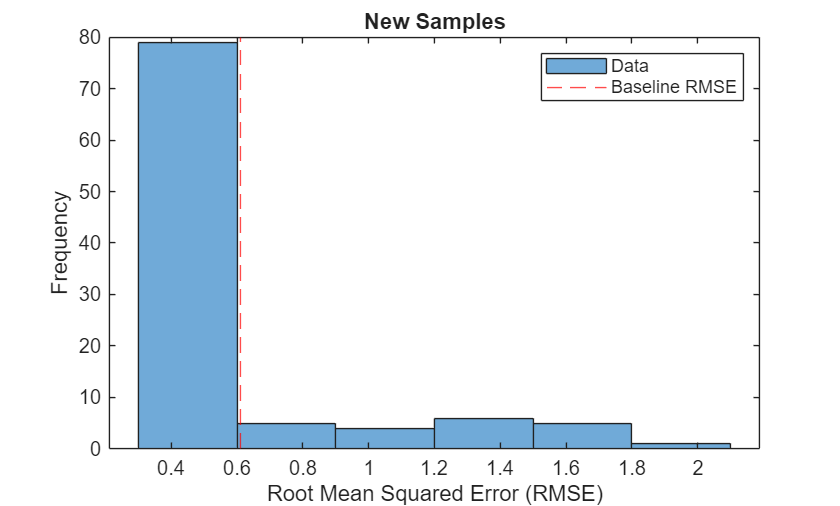### Identify Anomalous Regions

To detect anomalous regions in a sequence, find the MAE between the input sequence and the reconstructed sequence and highlight the regions with the error above a threshold value.

Calculate the error between the input sequence and the reconstructed sequence.

`MAE = mean(abs(Y - X),1);`

Set the time step window size to 7. Identify windows that have time steps with MAE values of at least 10% above the maximum error value identified using the validation data.

```windowSize = 7; thr = 1.1*MAEbaseline; idxAnomaly = false(1,size(X,2)); for t = 1:(size(X,2) - windowSize + 1) idxWindow = t:(t + windowSize - 1); if all(MAE(idxWindow) > thr) idxAnomaly(idxWindow) = true; end end```

Display the sequence in a plot and highlight the anomalous regions.

```figure t = tiledlayout(numChannels,1); title(t,"Anomaly Detection ") for i = 1:numChannels nexttile plot(X(i,:)); ylabel("Channel " + i) box off hold on XAnomalous = nan(1,size(X,2)); XAnomalous(idxAnomaly) = X(i,idxAnomaly); plot(XAnomalous,"r",LineWidth=3) hold off end xlabel("Time Step") nexttile(1) legend(["Input" "Anomalous"])```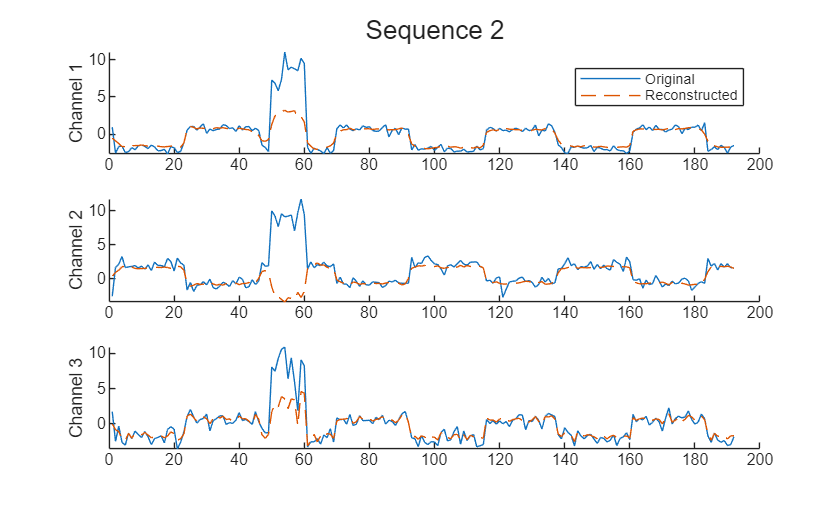The highlighted regions indicate the windows of time steps where the error values are at least 10% higher than the maximum error value.